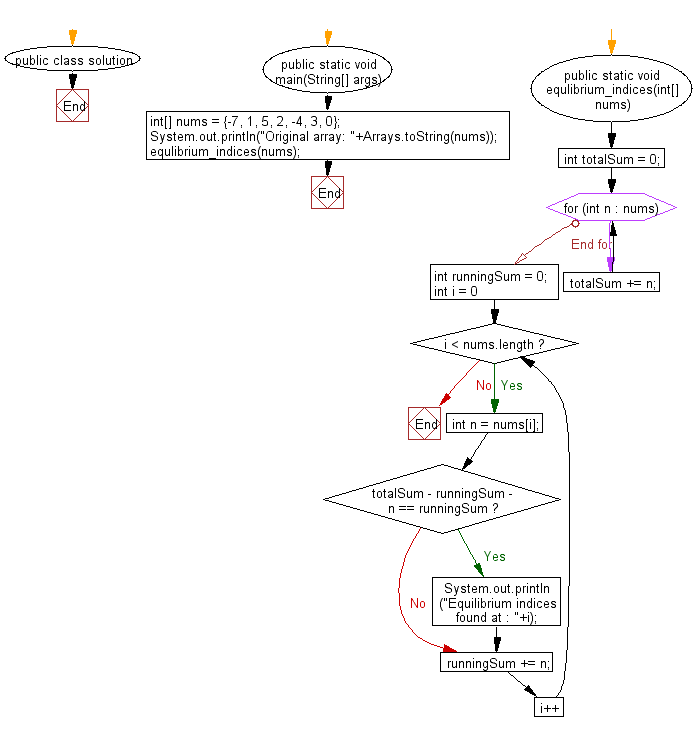﻿ Java: Find the equilibrium indices from an array of integers# Java Array Exercises: Find the equilibrium indices from a given array of integers

## Java Array: Exercise-62 with Solution

Write a Java program to find the equilibrium indices from a given array of integers.

An equilibrium index of a sequence is an index into the sequence such that the sum of elements at lower indices is equal to the sum of elements at higher indices.

```For example, in a sequence A:
A0 = -7
A1 = 1
A2 = 5
A3 = 2
A4 = -4
A5 = 3
A6 = 0
3 is an equilibrium index, because:

A0 + A1 + A2 = A4 + A5 + A6

6 is also an equilibrium index, because:

A0 + A1 + A2 + A3 + A4 + A5 = 0

(sum of zero elements is zero)

7 is not an equilibrium index, because it is not a valid index of sequence A.```

Example:
Input :
nums = {-7, 1, 5, 2, -4, 3, 0}
Output:
Equilibrium indices found at : 3
Equilibrium indices found at : 6
Source: https://bit.ly/2ziUROQ

Sample Solution:

Java Code:

``````import java.util.Arrays;
public class solution {
public static void main(String[] args) {
int[] nums = {-7, 1, 5, 2, -4, 3, 0};
System.out.println("Original array: "+Arrays.toString(nums));
equlibrium_indices(nums);
}

public static void equlibrium_indices(int[] nums){
//find total sum
int totalSum = 0;
for (int n : nums) {
totalSum += n;
}
//compare running sum to remaining sum to find equlibrium indices
int runningSum = 0;
for (int i = 0; i < nums.length; i++) {
int n = nums[i];
if (totalSum - runningSum - n == runningSum) {
System.out.println("Equilibrium indices found at : "+i);
}
runningSum += n;
}
}
}
```
```

Sample Output:

```Original array: [-7, 1, 5, 2, -4, 3, 0]
Equilibrium indices found at : 3
Equilibrium indices found at : 6
```

Flowchart:Java Code Editor:

Improve this sample solution and post your code through Disqus

What is the difficulty level of this exercise?

Test your Programming skills with w3resource's quiz.

﻿

## Java: Tips of the Day

countOccurrences

Counts the occurrences of a value in an array.

Use Arrays.stream().filter().count() to count total number of values that equals the specified value.

```public static long countOccurrences(int[] numbers, int value) {
return Arrays.stream(numbers)
.filter(number -> number == value)
.count();
}
```

Ref: https://bit.ly/3kCAgLb